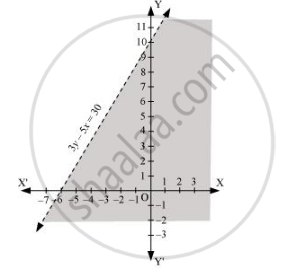CBSE (Arts) Class 11CBSE
Share

# Solve the Given Inequality Graphically in Two-dimensional Plane: 3y – 5x < 30 - CBSE (Arts) Class 11 - Mathematics

ConceptGraphical Solution of Linear Inequalities in Two Variables

#### Question

Solve the given inequality graphically in two-dimensional plane: 3y – 5x < 30

#### Solution

The graphical representation of 3y – 5x = 30 is given as dotted line in the figure below.

This line divides the xy-plane in two half planes.

Select a point (not on the line), which lies in one of the half planes, to determine whether the point satisfies the given inequality or not.

We select the point as (0, 0).

It is observed that,

3(0) – 5(0) < 30 or 0 < 30, which is true

Therefore, the upper half plane is not the solution region of the given inequality. Also, it is evident that any point on the line does not satisfy the given inequality.

Thus, the solution region of the given inequality is the half plane containing the point (0, 0) excluding the line.

The solution region is represented by the shaded region as follows.Is there an error in this question or solution?

#### Video TutorialsVIEW ALL 

Solution Solve the Given Inequality Graphically in Two-dimensional Plane: 3y – 5x < 30 Concept: Graphical Solution of Linear Inequalities in Two Variables.
S